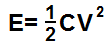﻿ Capacitor Energy Calculator

# Capacitor Energy CalculatorpC nC µC C

V

0.0000000000J

The capacitor energy calculator calculates the energy stored in a capacitor based on the size of the capacitance of the capacitor and the voltage that is dropped across the capacitor, according to the above formula.

A user enters the capacitance, C, and the voltage, V and the result will automatically be calculated and shown. The result which is displayed above is in unit Joules (J).

The energy stored in a capacitor is a very useful value to know, because in many circuits, capacitor's stored energy is an integral part to the functioning of a circuit. In many electronic circuits, capacitors store energy only later to be dispersed for the circuit to work. This is how flash cameras work. Capacitors store charge and then later dumps it all at once.

Related Resources